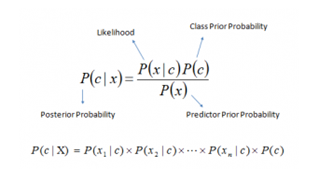In a world where many manual operations are mechanized, the definition of the word ‘manual’ is evolving. Computers can play chess, perform surgery, and develop into smarter, more humanlike machines with the aid of machine learning algorithms.

What is Naive Bayes Algorithm?

The machine learning algorithm is divided into categories, in which Naive Bayes Algorithm falls under the umbrella of supervised machine learning algorithm. It is a classification method built on the Bayes Theorem and predicated on the idea of predictor independence. A Naive Bayes classifier believes that the presence of one feature in a class has nothing to do with the presence of any other feature. For example

The Bayes model is simple to construct and especially helpful for very big data sets. Along with being straightforward, Naive Bayes is known to perform better than even the most complex classification techniques.

It provides a way of calculating the posterior probability by the given equation below:Where P(c|x) = posterior probability of class (c) given predictor (x).

P(x|c) = Prior probability of predictor.

Types of Naive Bayes Algorithm

Three different Naive Bayes model types may be found in the Scikit-Learn library which are as follows: -

Multinominal Naive Bayes

Feature vectors represent the frequencies with which certain events have been generated by a multinomial distribution. This is the event model that is typically used for document classification.

Bernoulli naive bayes:

In the multivariate Bernoulli event model, features are independent Booleans (binary variables) describing inputs. Like the multinomial model, this model is popular used for document classification tasks, where binary term occurrence (i.e., a word occurs in a document or not) features are used rather than term frequencies (i.e., frequency of a word in the document).

Gaussian Naive Bayes:

We assume that the values of the predictors are samples from a gaussian distribution when they take up a continuous value and are not discrete.

Below code can be implemented of Gaussian Naive Bayes classifier using scikit-learn.

 # load the iris dataset from sklearn.datasets import load_iris iris = load_iris()   # store the feature matrix (X) and response vector (y) X = iris.data y = iris.target   # splitting X and y into training and testing sets from sklearn.model_selection import train_test_split X_train, X_test, y_train, y_test = train_test_split(X, y, test_size=0.4, random_state=1)   # training the model on training set from sklearn.naive_bayes import GaussianNB gnb = GaussianNB() gnb.fit(X_train, y_train)   # making predictions on the testing set y_pred = gnb.predict(X_test)   # comparing actual response values (y_test) with predicted response values (y_pred) from sklearn import metrics print("Gaussian Naive Bayes model accuracy(in %):", metrics.accuracy_score(y_test, y_pred)*100)

Output:

Gaussian Naive Bayes model accuracy (in %): 95.0

Applications of Naive Bayes Algorithm/Classifier

Real-time Prediction: Naive Bayes is a quick classifier that is eager to learn. As a result, it might be applied to real-time prediction.

Multi-class Prediction: This algorithm is very widely renowned for its ability to predict many classes. Here, we can forecast the likelihood of several target variable classes.

Sentiment analysis, spam filtering, and text classification: Because they perform better in multi-class situations and follow the independence criterion, naive Bayes classifiers are frequently employed in text classification and have a greater success rate than other methods. It is therefore frequently used in Sentiment Analysis and Spam Filtering (to identify spam e-mail) (in social media analysis, to identify positive and negative customer sentiments)

Recommendation system:  Naive Bayes Classifier and Collaborative Filtering work together to create a system that filters opportunistic information and forecasts whether a user will find a specific resource appealing or not.